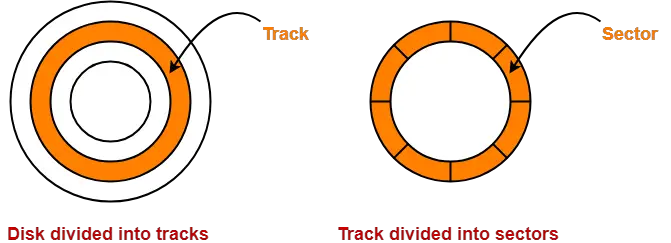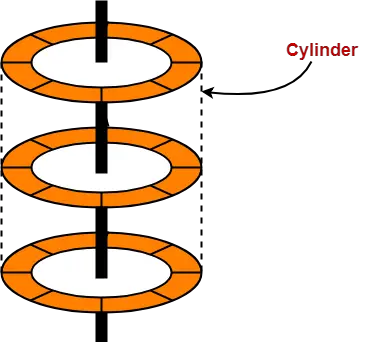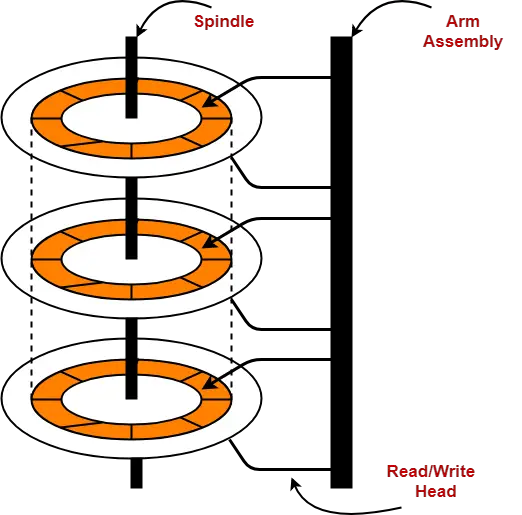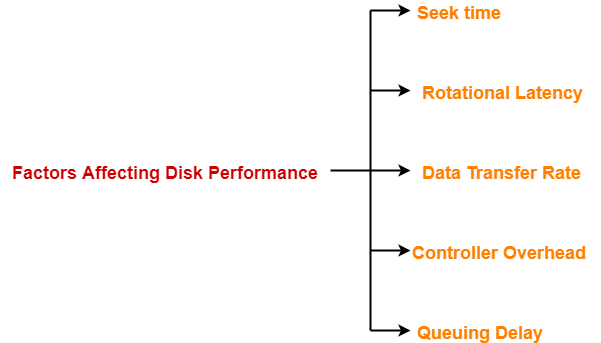## Magnetic Disk in Computer Architecture-

Before you go through this article, make sure that you have gone through the previous article on Magnetic Disk.

We have discussed-

• Magnetic disk is divided into platters which are further divided into tracks and sectors.
• Read / write head is a mechanical arm that is used to write to and read from the disk.
• Various important formulas.

In this article, we will discuss practice problems based on magnetic disk.

## Problem-01:

Consider a disk pack with the following specifications- 16 surfaces, 128 tracks per surface, 256 sectors per track and 512 bytes per sector.

1. What is the capacity of disk pack?
2. What is the number of bits required to address the sector?
3. If the format overhead is 32 bytes per sector, what is the formatted disk space?
4. If the format overhead is 64 bytes per sector, how much amount of memory is lost due to formatting?
5. If the diameter of innermost track is 21 cm, what is the maximum recording density?
6. If the diameter of innermost track is 21 cm with 2 KB/cm, what is the capacity of one track?
7. If the disk is rotating at 3600 RPM, what is the data transfer rate?
8. If the disk system has rotational speed of 3000 RPM, what is the average access time with a seek time of 11.5 msec?

## Solution-

Given-

• Number of surfaces = 16
• Number of tracks per surface = 128
• Number of sectors per track = 256
• Number of bytes per sector = 512 bytes

### Part-01: Capacity of Disk Pack-

Capacity of disk pack

= Total number of surfaces x Number of tracks per surface x Number of sectors per track x Number of bytes per sector

= 16 x 128 x 256 x 512 bytes

= 228 bytes

= 256 MB

### Part-02: Number of Bits Required To Address Sector-

Total number of sectors

= Total number of surfaces x Number of tracks per surface x Number of sectors per track

= 16 x 128 x 256 sectors

= 219 sectors

Thus, Number of bits required to address the sector = 19 bits

### Part-03: Formatted Disk Space-

= Total number of sectors x overhead per sector

= 219 x 32 bytes

= 219 x 25 bytes

= 224 bytes

= 16 MB

Now, Formatted disk space

= Total disk space – Formatting overhead

= 256 MB – 16 MB

= 240 MB

Amount of memory lost due to formatting

= Total number of sectors x Overhead per sector

= 219 x 64 bytes

= 219 x 26 bytes

= 225 bytes

= 32 MB

### Part-05: Maximum Recording Density-

Storage capacity of a track

= Number of sectors per track x Number of bytes per sector

= 256 x 512 bytes

= 28 x 29 bytes

= 217 bytes

= 128 KB

Circumference of innermost track

= 2 x π x radius

= π x diameter

= 3.14 x 21 cm

= 65.94 cm

Now, Maximum recording density

= Recording density of innermost track

= Capacity of a track / Circumference of innermost track

= 128 KB / 65.94 cm

= 1.94 KB/cm

### Part-06: Capacity Of Track-

Circumference of innermost track

= 2 x π x radius

= π x diameter

= 3.14 x 21 cm

= 65.94 cm

Capacity of a track

= Storage density of the innermost track x Circumference of the innermost track

= 2 KB/cm x 65.94 cm

= 131.88 KB

≅ 132 KB

### Part-07: Data Transfer Rate-

Number of rotations in one second

= (3600 / 60) rotations/sec

= 60 rotations/sec

Now, Data transfer rate

= Number of heads x Capacity of one track x Number of rotations in one second

= 16 x (256 x 512 bytes) x 60

= 24 x 28 x 29 x 60 bytes/sec

= 60 x 221 bytes/sec

= 120 MBps

### Part-08: Average Access Time-

Time taken for one full rotation

= (60 / 3000) sec

= (1 / 50) sec

= 0.02 sec

= 20 msec

Average rotational delay

= 1/2 x Time taken for one full rotation

= 1/2 x 20 msec

= 10 msec

Now, average access time

= Average seek time + Average rotational delay + Other factors

= 11.5 msec + 10 msec + 0

= 21.5 msec

## Problem-02:

What is the average access time for transferring 512 bytes of data with the following specifications-

• Average seek time = 5 msec
• Disk rotation = 6000 RPM
• Data rate = 40 KB/sec
• Controller overhead = 0.1 msec

## Solution-

Given-

• Average seek time = 5 msec
• Disk rotation = 6000 RPM
• Data rate = 40 KB/sec
• Controller overhead = 0.1 msec

### Time Taken For One Full Rotation-

Time taken for one full rotation

= (60 / 6000) sec

= (1 / 100) sec

= 0.01 sec

= 10 msec

### Average Rotational Delay-

Average rotational delay

= 1/2 x Time taken for one full rotation

= 1/2 x 10 msec

= 5 msec

### Transfer Time-

Transfer time

= (512 bytes / 40 KB) sec

= 0.0125 sec

= 12.5 msec

### Average Access Time-

Average access time

= Average seek time + Average rotational delay + Transfer time + Controller overhead + Queuing delay

= 5 msec + 5 msec + 12.5 msec + 0.1 msec + 0

= 22.6 msec

## Problem-03:

A certain moving arm disk storage with one head has the following specifications-

• Number of tracks per surface = 200
• Disk rotation speed = 2400 RPM
• Track storage capacity = 62500 bits
• Average latency = P msec
• Data transfer rate = Q bits/sec

What is the value of P and Q?

## Solution-

Given-

• Number of tracks per surface = 200
• Disk rotation speed = 2400 RPM
• Track storage capacity = 62500 bits

### Time Taken For One Full Rotation-

Time taken for one full rotation

= (60 / 2400) sec

= (1 / 40) sec

= 0.025 sec

= 25 msec

### Average Latency-

Average latency or Average rotational latency

= 1/2 x Time taken for one full rotation

= 1/2 x 25 msec

= 12.5 msec

### Data Transfer Rate-

Data transfer rate

= Number of heads x Capacity of one track x Number of rotations in one second

= 1 x 62500 bits x (2400 / 60)

= 2500000 bits/sec

= 2.5 x 106 bits/sec

Thus, P = 12.5 and Q = 2.5 x 106

## Problem-04:

A disk pack has 19 surfaces and storage area on each surface has an outer diameter of 33 cm and inner diameter of 22 cm. The maximum recording storage density on any track is 200 bits/cm and minimum spacing between tracks is 0.25 mm. Calculate the capacity of disk pack.

## Solution-

Given-

• Number of surfaces = 19
• Outer diameter = 33 cm
• Inner diameter = 22 cm
• Maximum recording density = 200 bits/cm
• Inter track gap = 0.25 mm

### Number Of Tracks On Each Surface-

Number of tracks on each surface

= (16.5 cm – 11 cm) / 0.25 mm

= 5.5 cm / 0.25 mm

= 55 mm / 0.25 mm

= 220 tracks

### Capacity Of Each Track-

Capacity of each track

= Maximum recording density x Circumference of innermost track

= 200 bits/cm x (3.14 x 22 cm)

= 200 x 69.08 bits

= 13816 bits

= 1727 bytes

### Capacity Of Disk Pack-

Capacity of disk pack

= Total number of surfaces x Number of tracks per surface x Capacity of one track

= 19 x 220 x 1727 bytes

= 7218860 bytes

= 6.88 MB

## Problem-05:

Consider a typical disk that rotates at 15000 RPM and has a transfer rate of 50 x 106 bytes/sec. If the average seek time of the disk is twice the average rotational delay and the controller’s transfer time is 10 times the disk transfer time. What is the average time (in milliseconds) to read or write a 512 byte sector of the disk?

## Solution-

Given-

• Rotation speed of the disk = 15000 RPM
• Transfer rate = 50 x 106 bytes/sec
• Average seek time = 2 x Average rotational delay
• Controller’s transfer time = 10 x Disk transfer time

### Time Taken For One Full Rotation-

Time taken for one full rotation

= (60 / 15000) sec

= 0.004 sec

= 4 msec

### Average Rotational Delay-

Average rotational delay

= 1/2 x Time taken for one full rotation

= 1/2 x 4 msec

= 2 msec

### Average Seek Time-

Average seek time

= 2 x Average rotational delay

= 2 x 2 msec

= 4 msec

### Disk Transfer Time-

Disk transfer time

= Time taken to read or write 512 bytes

= 512 bytes / (50 x 106 bytes/sec)

= 10.24 x 10-6 sec

= 0.01024 msec

### Controller’s Transfer Time-

Controller’s transfer time

= 10 x Disk transfer time

= 10 x 0.01024 msec

= 0.1024 msec

### Average Time To Read Or Write 512 Bytes-

Average time to read or write 512 bytes

= Average seek time + Average rotational delay + Disk transfer time + Controller’s transfer time + Queuing delay

= 4 msec + 2 msec + 0.01024 msec + 0.1024 msec + 0

= 6.11 msec

## Problem-06:

A hard disk system has the following parameters-

• Number of tracks = 500
• Number of sectors per track = 100
• Number of bytes per sector = 500
• Time taken by the head to move from one track to another adjacent track = 1 msec
• Rotation speed = 600 RPM

What is the average time taken for transferring 250 bytes from the disk?

## Solution-

Given-

• Number of tracks = 500
• Number of sectors per track = 100
• Number of bytes per sector = 500
• Time taken by the head to move from one track to another adjacent track = 1 msec
• Rotation speed = 600 RPM

### Average Seek Time-

Average seek time

= (Time taken by the head to move from track-1 to track-1 + Time taken by the head to move from track-1 to track-500) / 2

= (0 + 499 x 1 msec) / 2

= 249.5 msec

### Time Taken For One Full Rotation-

Time taken for one full rotation

= (60 / 600) sec

= 0.1 sec

= 100 msec

### Average Rotational Delay-

Average rotational delay

= 1/2 x Time taken for one full rotation

= 1/2 x 100 msec

= 50 msec

### Capacity Of One Track-

Capacity of one track

= Number of sectors per track x Number of bytes per sector

= 100 x 500 bytes

= 50000 bytes

### Data Transfer Rate-

Data transfer rate

= Number of heads x Capacity of one track x Number of rotations in one second

= 1 x 50000 bytes x (600 / 60)

= 50000 x 10 bytes/sec

= 5 x 105 bytes/sec

### Transfer Time-

Transfer time

= (250 bytes / 5 x 105 bytes) sec

= 50 x 10-5 sec

= 0.5 msec

### Average Time Taken To Transfer 250 Bytes-

Average time taken to transfer 250 bytes

= Average seek time + Average rotational delay + Transfer time + Controller overhead + Queuing delay

= 249.5 msec + 50 msec + 0.5 msec + 0 + 0

= 300 msec

## Problem-07:

A hard disk has 63 sectors per track, 10 platters each with 2 recording surfaces and 1000 cylinders.

The address of a sector is given as a triple (c, h, s) where c is the cylinder number, h is the surface number and s is the sector number. Thus, the 0th sector is addressed as (0,0,0), the 1st sector as (0,0,1) and so on.

### Part-01:

The address <400, 16, 29> corresponds to sector number-

1. 505035
2. 505036
3. 505037
4. 505038

### Part-02:

The address of 1039 sector is-

1. <0, 15, 31>
2. <0, 16, 30>
3. <0, 16, 31>
4. <0, 17, 31>

## Solution-

### Know this Concept?

In general, when counting of items is started from 0, then-

• For any item-n, number ‘n’ specifies the number of items that must be crossed in order to reach that item.

### Example-

If counting is started from 0, then-

• To reach cylinder-5, the number of cylinders that must be crossed = 5 cylinders
• To reach surface-5, the number of surfaces that must be crossed = 5 surfaces
• To reach sector-5, the number of sectors that must be crossed = 5 sectors

To solve this question, we assume there is only one track on each surface.

## Part-01:

We have to calculate the sector number for the address <400, 16, 29>

### Step-01:

To reach our desired cylinder, we have to cross 400 cylinders.

Total number of sectors that are crossed in 400 cylinders

= Number of cylinders x Number of surfaces per cylinder x Number of tracks per surface x Number of sectors per track

= 400 x (10 x 2) x 1 x 63

= 504000

Now, after crossing 400 cylinders (cylinder-0 to cylinder-399), we are at cylinder-400.

### Step-02:

To reach our desired surface, we have to cross 16 surfaces.

Total number of sectors that are crossed in 16 surfaces

= Number of surfaces x Number of tracks per surface x Number of sectors per track

= 16 x 1 x 63

= 1008

Now, after crossing 16 surfaces (surface-0 to surface-15) in cylinder-400, we are at surface-16.

### Step-03:

To reach our desired sector, we have to cross 29 sectors.

Now, after crossing 29 sectors on surface-16 of cylinder-400, we are at sector-29.

Thus

Total number of sectors that are crossed

= 504000 + 1008 + 29

= 505037

Thus,

• After crossing 505037 sectors, we are at sector-505037.
• So, required address of the sector is 505037.
• Option (C) is correct.

## Part-02:

We have to find the address of the sector-2039.

Let us check all the options one by one.

### Option-A:

For the address <0, 15, 31>, the sector number is-

Sector number = 0 + (15 x 1 x 63) + 31 = 976

### Option-B:

For the address <0, 16, 30>, the sector number is-

Sector number = 0 + (16 x 1 x 63) + 30 = 1038

### Option-C:

For the address <0, 16, 31>, the sector number is-

Sector number = 0 + (16 x 1 x 63) + 31 = 1039

### Option-D:

For the address <0, 17, 31>, the sector number is-

Sector number = 0 + (17 x 1 x 63) + 31 = 1102

Thus, Option (C) is correct.

Get more notes and other study material of Computer Organization and Architecture.

Watch video lectures by visiting our YouTube channel LearnVidFun.

## Magnetic Disk in Computer Architecture-

In computer architecture,

• Magnetic disk is a storage device that is used to write, rewrite and access data.
• It uses a magnetization process.

## Architecture-

• The entire disk is divided into platters.
• Each platter consists of concentric circles called as tracks.
• These tracks are further divided into sectors which are the smallest divisions in the disk.• A cylinder is formed by combining the tracks at a given radius of a disk pack.• There exists a mechanical arm called as Read / Write head.
• It is used to read from and write to the disk.
• Head has to reach at a particular track and then wait for the rotation of the platter.
• The rotation causes the required sector of the track to come under the head.
• Each platter has 2 surfaces- top and bottom and both the surfaces are used to store the data.## Disk Performance Parameters-

The time taken by the disk to complete an I/O request is called as disk service time or disk access time.

Components that contribute to the service time are-1. Seek time
2. Rotational latency
3. Data transfer rate
5. Queuing delay

## 1. Seek Time-

• The time taken by the read / write head to reach the desired track is called as seek time.
• It is the component which contributes the largest percentage of the disk service time.
• The lower the seek time, the faster the I/O operation.

## Specifications

Seek time specifications include-

1. Full stroke
2. Average
3. Track to Track

### 1. Full Stroke-

• It is the time taken by the read / write head to move across the entire width of the disk from the innermost track to the outermost track

### 2. Average-

• It is the average time taken by the read / write head to move from one random track to another.

 Average seek time = 1 / 3 x Full stroke

## 2. Rotational Latency-

• The time taken by the desired sector to come under the read / write head is called as rotational latency.
• It depends on the rotation speed of the spindle.

 Average rotational latency = 1 / 2 x Time taken for full rotation

## 3. Data Transfer Rate-

• The amount of data that passes under the read / write head in a given amount of time is called as data transfer rate.
• The time taken to transfer the data is called as transfer time.

It depends on the following factors-

1. Number of bytes to be transferred
2. Rotation speed of the disk
3. Density of the track
4. Speed of the electronics that connects the disk to the computer

• The overhead imposed by the disk controller is called as controller overhead.
• Disk controller is a device that manages the disk.

## 5. Queuing Delay-

• The time spent waiting for the disk to become free is called as queuing delay.

## NOTE-

 All the tracks of a disk have the same storage capacity.

## Storage Density-

• All the tracks of a disk have the same storage capacity.
• This is because each track has different storage density.
• Storage density decreases as we from one track to another track away from the center.

Thus,

• Innermost track has maximum storage density.
• Outermost track has minimum storage density.

## Important Formulas-

### 1. Disk Access Time-

Disk access time is calculated as-

 Disk access time = Seek time + Rotational delay + Transfer time + Controller overhead + Queuing delay

### 2. Average Disk Access Time-

Average disk access time is calculated as-

 Average disk access time = Average seek time + Average rotational delay + Transfer time + Controller overhead + Queuing delay

### 3. Average Seek Time-

Average seek time is calculated as-

 Average seek time = 1 / 3 x Time taken for one full stroke

Alternatively,

If time taken by the head to move from one track to adjacent track = t units and there are total k tracks, then-

Average seek time

= { Time taken to move from track 1 to track 1 + Time taken to move from track 1 to last track } / 2

= { 0 + (k-1)t } / 2

= (k-1)t / 2

### 4. Average Rotational Latency-

Average rotational latency is calculated as-

 Average rotational latency = 1 / 2 x Time taken for one full rotation

Average rotational latency may also be referred as-

• Average rotational delay
• Average latency
• Average delay

### 5. Capacity Of Disk Pack-

Capacity of a disk pack is calculated as-

 Capacity of a disk pack = Total number of surfaces x Number of tracks per surface x Number of sectors per track x Storage capacity of one sector

### 7. Formatted Disk Space-

Formatted disk space also called as usable disk space is the disk space excluding formatting overhead.

It is calculated as-

 Formatted disk space = Total disk space or capacity – Formatting overhead

### 8. Recording Density Or Storage Density-

Recording density or Storage density is calculated as-

 Storage density of a track = Capacity of the track / Circumference of the track

From here, we can infer-

Storage density of a track ∝ 1 / Circumference of the track

### 9. Track Capacity-

Capacity of a track is calculated as-

 Capacity of a track = Recording density of the track x Circumference of the track

### 10. Data Transfer Rate-

Data transfer rate is calculated as-

 Data transfer rate = Number of heads x Bytes that can be read in one full rotation x Number of rotations in one second

OR

 Data transfer rate = Number of heads x Capacity of one track x Number of rotations in one second

### 11. Tracks Per Surface-

Total number of tracks per surface is calculated as-

 Total number of tracks per surface = (Outer radius – Inner radius) / Inter track gap

## Points to Remember-

• The entire disk space is not usable for storage because some space is wasted in formatting.
• When rotational latency is not given, use average rotational latency for solving numerical problems.
• When seek time is not given, use average seek time for solving numerical problems.
• It is wrong to say that as we move from one track to another away from the center, the capacity increases.
• All the tracks have same storage capacity.

To gain better understanding about magnetic disk-

Watch this Video Lecture

Next Article- Practice Problems On Magnetic Disk

Get more notes and other study material of Computer Organization and Architecture.

Watch video lectures by visiting our YouTube channel LearnVidFun.